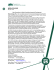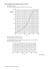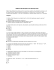Economics 151 Development Economics Sample Exam Questions

Transcription

Economics 151 Development Economics Sample Exam Questions
```Economics 151
Development Economics
Sample Exam Questions
Circle one answer only for each multiple choice question. Each question is worth 2½
points.
1. By the World Bank’s classification system, Malaysia, Mexico and Brazil are
a. low-income countries
b. upper-middle-income countries
c. industrial economies
d. underdeveloped countries
2. In 1997, the World Bank found that the fraction of the world’s population living in
low and lower-middle income countries—that is, countries with per capita incomes of
\$1230 or less—was approximately
a.
b.
c.
d.
25%
40%
55%
75%
3. Overall, the growth rate of average incomes in less developed countries between 1960
and 1995
a.
b.
c.
d.
was approximately zero
exceeded that of high income countries
exceeded that of Britain during the industrial revolution
was approximately 3.0% per year
4. Most Latin American countries achieved independence
a.
b.
c.
d.
shortly after World War II
in the 1960s
around the time of World War I
in the early nineteenth century
1
5. Many countries that became independent after World War II found that the legacy of
colonialism hindered their economic development. According to the textbook, the list
of hindrances included
a. artificial political boundaries that covered diverse ethnic groups with little in
common
c. control of domestic commerce by foreign minority groups which had been
brought in by the colonial power
d. all of the above
6. At the time of independence, there were already hundreds of thousands of university
graduates in ____, but hardly any at all in ____.
a.
b.
c.
d.
India, Congo
India, South Korea
Congo, South Korea
South Korea, India
7. Which of the following is not viewed by the text as a major political obstacle to
development?
a.
b.
c.
d.
growth oriented policies may damage the short-term interests of influential groups
civil war
corruption
departing from strict laissez faire principles
8. In the Harrod-Domar equation g = s/v, v is defined as
a.
b.
c.
d.
the value of the country’s capital stock
the ratio of the country’s capital stock to its output
the change in the country’s capital stock
none of the above
9. A sources of growth equation derived from the neoclassical production function Y =
f(K,L,R,A) is gY = a + wKgK + wLgL + wRgR. In this equation, gK refers to the growth
rate of the capital stock and wK is
a.
b.
c.
d.
the average wage of capital owners
a weight on capital typically set at the rate of interest
a weight on capital typically set to 1/3 so that wK + wL + wR = 1.
a weight on capital typically set equal to capital’s share in national income
2
10. Suppose that in the equation above gY = .04, that gK, gL, and gR are all = .03, and wK
+ wL + wR = 1. What does this imply?
a.
b.
c.
d.
national income must be growing by 7% a year
national income must be growing by 1% a year
productivity must be growing by 1% a year
productivity must be growing by 7% a year
11. In the three-part diagram of the labor-surplus model in Figure 3-5, the marginal
product of labor in the agricultural sector can be measured in the lower panel (A) by
a. the height the solid curve at a given quantity of labor
b. the angle of a ray from the zero point to the solid curve at a given quantity of
labor
c. the length on the (bottom) horizontal axis from the zero point to the quantity of
labor in question
d. the (negative of the) slope of the solid curve at a given quantity of labor
12. If the number of agricultural workers is i, a commercial (i.e., profit maximizing)
farmer would find it profitable to hire a laborer
a.
b.
c.
d.
as long as the wage is less than the distance t’h (shown in the middle panel)
as long as the wage is less than the distance t’’k (shown in the top panel)
only under a share-cropping arrangement
13. World Bank data show that in 1995, the poorest 20% of households accounted for
7.5% of household income in Niger, the next 20% of households accounted for 11.8%
of income, the middle 20% accounted for 15.5% of income, the second richest 20%
accounted for 21.1% of income, and the top 20% accounted for 44.1% of income.
What was the cumulative income share of the bottom 60% of households in Niger?
a.
b.
c.
d.
15.5%
34.8%
48.1%
65.2%
14. Figures a, b, and c represent Lorenz curves for the distribution of income in various
countries. Which curve corresponds most closely to the data given for Niger in the
question above?
a. Figure a
b. Figure b
c. Figure c
3
Figure a
100
cumulative
percent
of income
80
60
40
20
0
0
20 40 60 80 100
cumulative percent of households
Figure b
100
cumulative
percent
of income
80
60
40
20
0
0
20 40 60 80 100
cumulative percent of households
Figure c
100
cumulative
percent
of income
80
60
40
20
0
0
20 40 60 80 100
cumulative percent of households
4
15. The Gini coefficient of income distribution in Niger was roughly:
a.
b.
c.
d.
.22
.36
.49
.61
16. The conjecture that inequality first increases with development, then decreases with
further development (known as the “inverted U hypothesis”) has been
a.
b.
c.
d.
strongly supported by most studies
supported mainly by cross-section, not time-series studies
supported mainly by time-series, not cross-section studies
generally repudiated by empirical studies
17. Which of the following is not an element of the redistribution-with-growth policy
approach?
a.
b.
c.
d.
minimum wage legislation
land reform
progressive taxation
18. Each of the following is listed by the textbook as one of the foundations for a wellfunctioning market economy, except:
a.
b.
c.
d.
stabilize macroeconomic conditions
establish a stock market
let relative prices reflect scarcity values
19. According to the input-output table of Table 3-1 (refer to textbook), the value of
services in dollars used directly to produce each dollar of manufactured consumer
goods is
____
5
20. The total revenue from operating a fishery is graphed against the level of fishing
effort as the curve TR shown in the figure below. The total cost of fishing as a
function of the effort expended is graphed as the line TC. Maximum net revenue,
attained at point ___, is/is not expected to result if the industry consists of large
numbers of unregulated fishermen each pursuing maximum income without
coordination with the others.
a.
b.
c.
d.
E*, is
E2, is not
E1, is
E*, is not
TC
Total Revenue
and
Total Cost
TR
E*
E2
E1 Effort
21. The curve S = PMC in the figure below shows the private marginal cost of producing
a certain manufactured good as a function of the quantity produced. The line D is the
demand curve for the good. Suppose that production of the good imposes a cost E
per unit on the environment which is not borne by the producing companies. Then an
appropriate label for the curve BB’ would be ______, the level of production without
government intervention would be ___, and the socially optimal level of production
would be ____.
a.
b.
c.
d.
External cost; Q2; Q1
External cost; Q1; Q2
Social marginal cost; Q2; Q1
Social marginal cost; Q1; Q2
Price
B’
S = PMC
B
E
D
Q2
Q1
Quantity
6
1. b
2. d
3. c
4. d
5. d
6. a
7. d
8. b
9. d
10. c
11. d
12. a
13. b
14. a
15. b
16. b
17. a
18. b
19. \$15/\$290 = \$0.05
20. d
21. d
7
```

Parabolic CurveA 30.00 milliliter sample of a weak monoprotic acid was... solution of NaOH.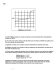Document 6523885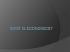3 sample exam paper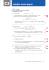[SAMPLE] [FATE] Admission Test MS/MPhil Applied Economics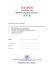Circular Flow Chart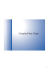Surfwood Manor apartments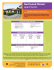IWRM – NEIAFU, VAVA`U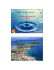WORKSHEET ECONOMICS CLASS XII SAMPLE PAPER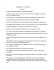Study Unit 2 - CMAPrepCourse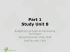Economics 2301-03 SAMPLE QUESTIONS FOR MIDTERM EXAM #2 Fall, 2011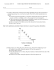Supply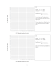Math 1172 Sample Test #1 + 1 and the line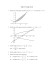Ballasted R40 Curve Baseplates - L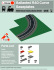lesto - Dux Lighting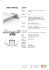Document 6537674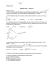Test 1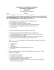Solutions for Econ 290 Sample Midterm One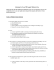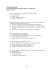CCIRC 5 May 2015 - Pacific Institute for Climate Solutions